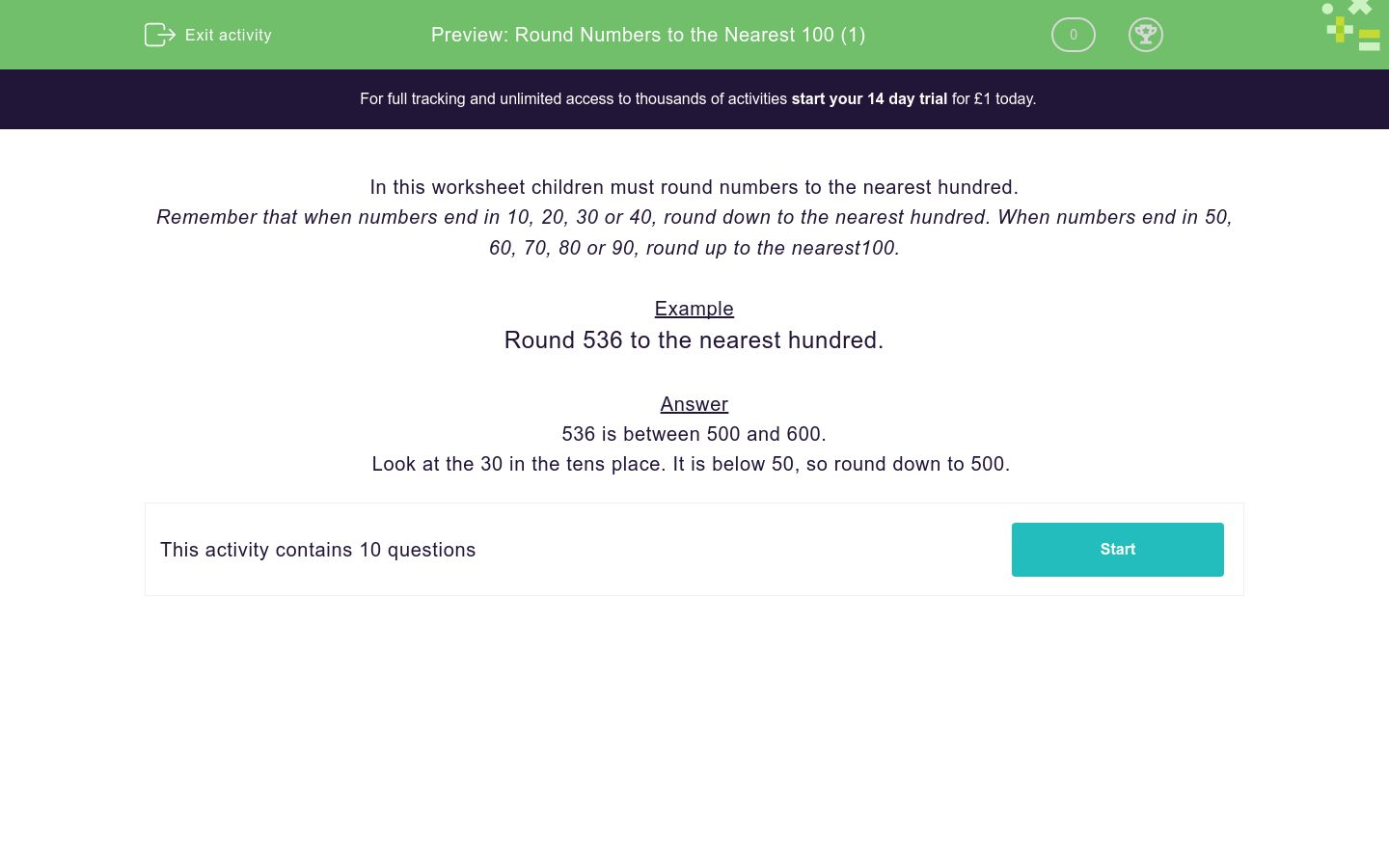# Round Numbers to the Nearest 100 (1)

In this worksheet, students round numbers to the nearest hundred.Key stage:  KS 2

Curriculum topic:   Number: Number and Place Value

Curriculum subtopic:   Round Numbers Up/Down (to 10, 100 or 1000)

Difficulty level:### QUESTION 1 of 10

In this worksheet children must round numbers to the nearest hundred.

Remember that when numbers end in 10, 20, 30 or 40, round down to the nearest hundred. When numbers end in 50, 60, 70, 80 or 90, round up to the nearest100.

Example

Round 536 to the nearest hundred.

536 is between 500 and 600.

Look at the 30 in the tens place. It is below 50, so round down to 500.

Round the number to the nearest hundred.

384

Round the number to the nearest hundred.

870

Round the number to the nearest hundred.

669

Round the number to the nearest hundred.

268

Round the number to the nearest hundred.

416

Round the number to the nearest hundred.

443

Round the number to the nearest hundred.

550

Round the number to the nearest hundred.

552

Round the number to the nearest hundred.

750

Round the number to the nearest hundred.

748

• Question 1

Round the number to the nearest hundred.

384

400
EDDIE SAYS
384 is between 300 and 400. Look at the 80 in the tens place. It is above 50, so round up to 400.
• Question 2

Round the number to the nearest hundred.

870

900
EDDIE SAYS
870 is between 800 and 900. Look at the 70 in the tens place. It is above 50, so round up to 900.
• Question 3

Round the number to the nearest hundred.

669

700
EDDIE SAYS
669 is between 600 and 700. Look at the 60 in the tens place. It is above 50, so round up to 700.
• Question 4

Round the number to the nearest hundred.

268

300
EDDIE SAYS
268 is between 200 and 300. Look at the 60 in the tens place. It is above 50, so round up to 300.
• Question 5

Round the number to the nearest hundred.

416

400
EDDIE SAYS
416 is between 400 and 500. Look at the 10 in the tens place. It is below 50, so round down to 400.
• Question 6

Round the number to the nearest hundred.

443

400
EDDIE SAYS
443 is between 400 and 500. Look at the 40 in the tens place. It is below 50, so round down to 400.
• Question 7

Round the number to the nearest hundred.

550

600
EDDIE SAYS
550 is between 500 and 600. Look at the 50 in the tens place and round up to 600.
• Question 8

Round the number to the nearest hundred.

552

600
EDDIE SAYS
552 is between 500 and 600. Look at the 50 in the tens place and round up to 600.
• Question 9

Round the number to the nearest hundred.

750

800
EDDIE SAYS
750 is between 700 and 800. Look at the 50 in the tens place and round up to 800.
• Question 10

Round the number to the nearest hundred.

748

700
EDDIE SAYS
748 is between 700 and 800. Look at the 40 in the tens place. It is below 50, so round down to 700.
---- OR ----

Sign up for a £1 trial so you can track and measure your child's progress on this activity.

### What is EdPlace?

We're your National Curriculum aligned online education content provider helping each child succeed in English, maths and science from year 1 to GCSE. With an EdPlace account you’ll be able to track and measure progress, helping each child achieve their best. We build confidence and attainment by personalising each child’s learning at a level that suits them.

Get started